# RS Aggarwal Solutions for Class 9 Chapter 12: Circles Exercise 12A

## RS Aggarwal Solutions for Class 9 Maths Exercise 12A PDF

RS Aggarwal solutions for Class 9 are prepared by highly experienced faculty at BYJU’S with 100% accuracy. The students while solving problems using RS Aggarwal textbook can download the PDF for free and refer them. The solutions are prepared with the objective of helping the students score well in the board exams. To make Mathematics simple for the students is the main aim of our faculty. This exercise of chapter 12 explains the congruence of circles and methods of solving problems based on them. RS Aggarwal Solutions for Class 9 Maths Chapter 12 Circles Exercise 12A are provided here.

## RS Aggarwal Solutions for Class 9 Chapter 12: Circles Exercise 12A Download PDF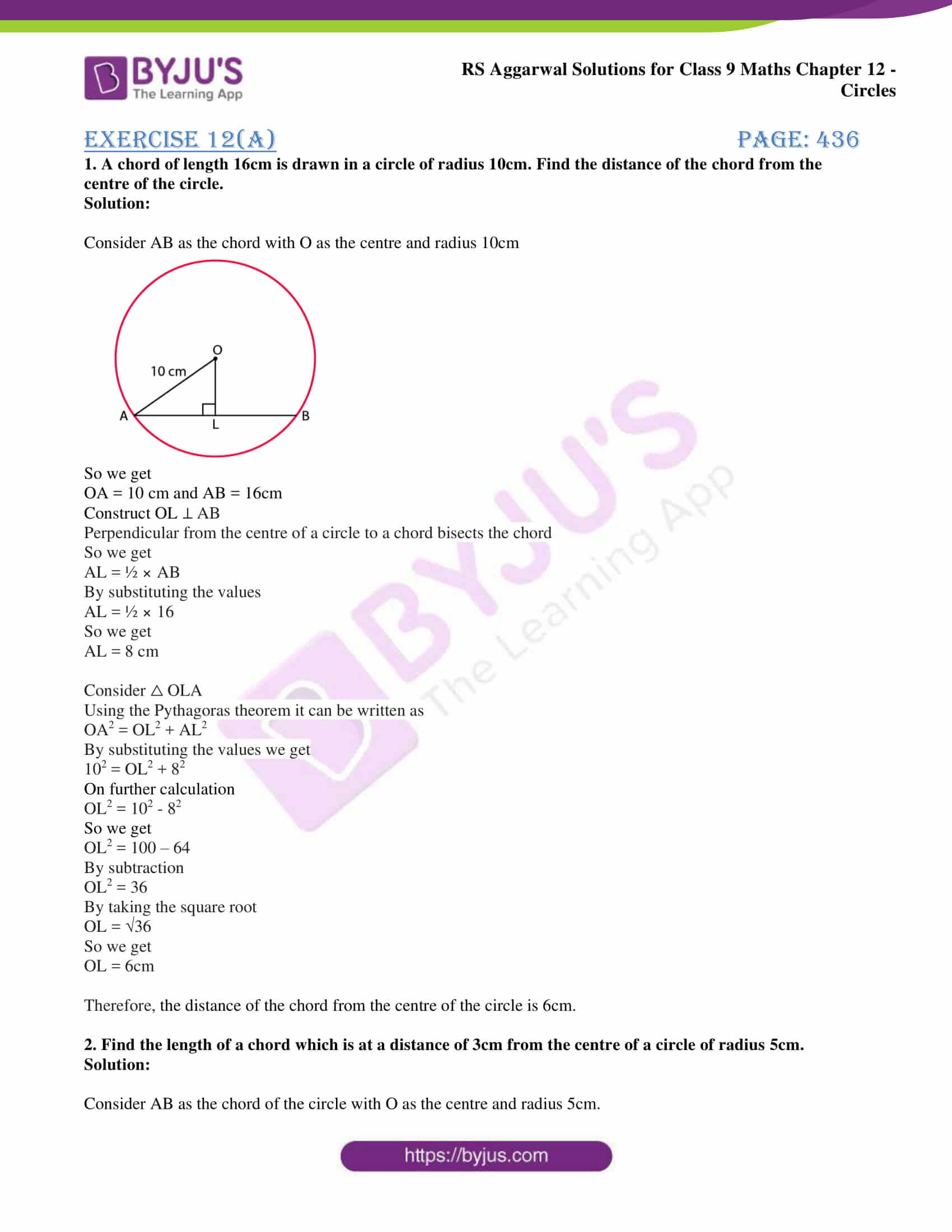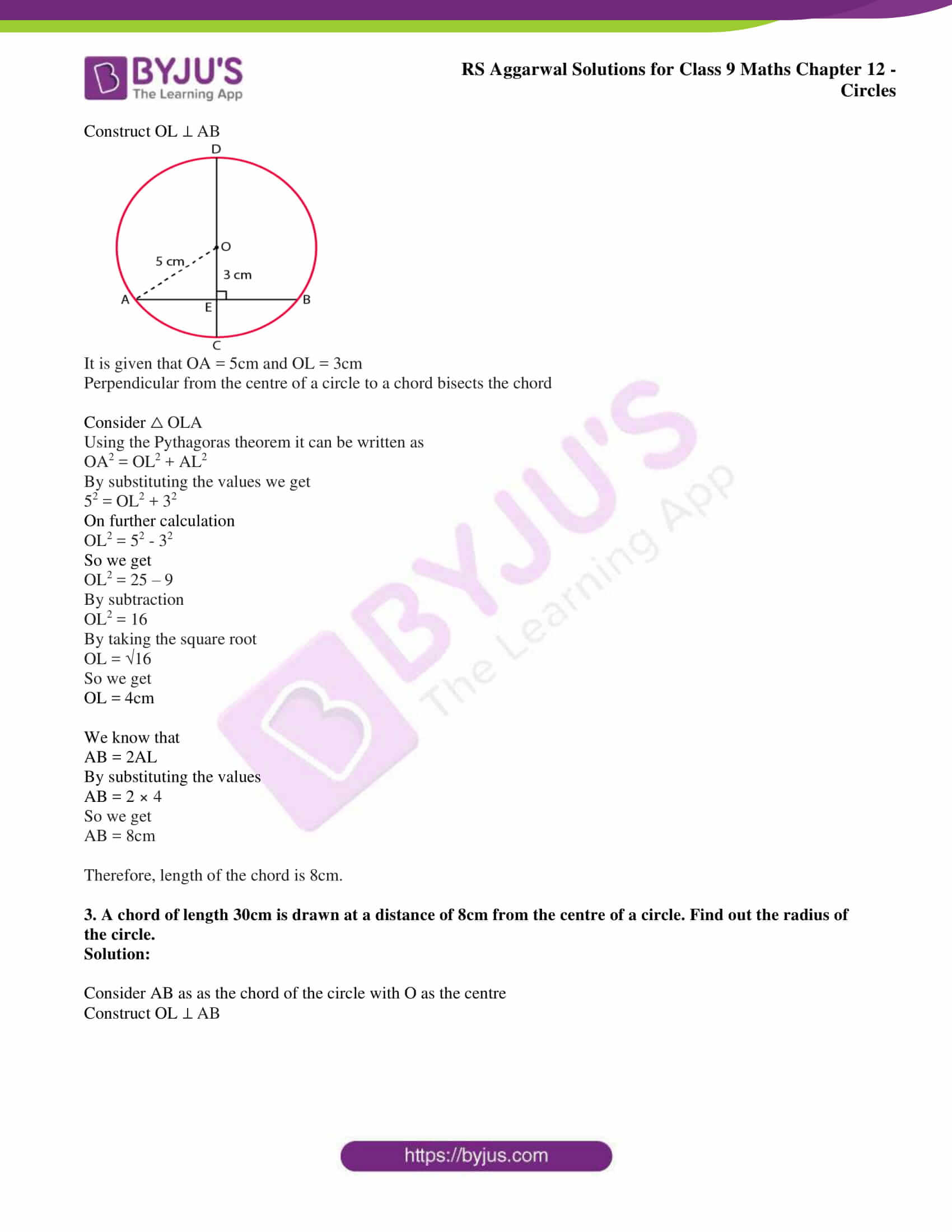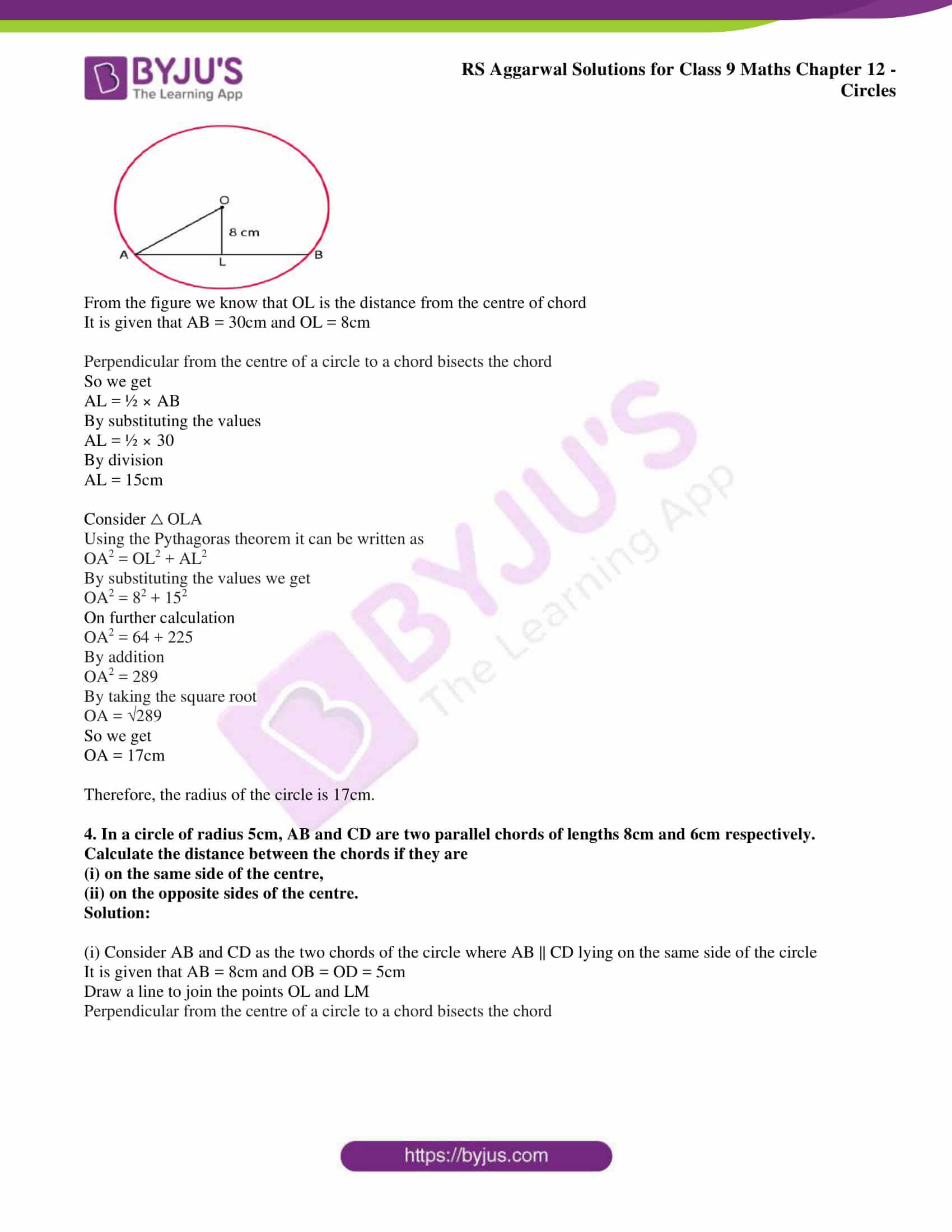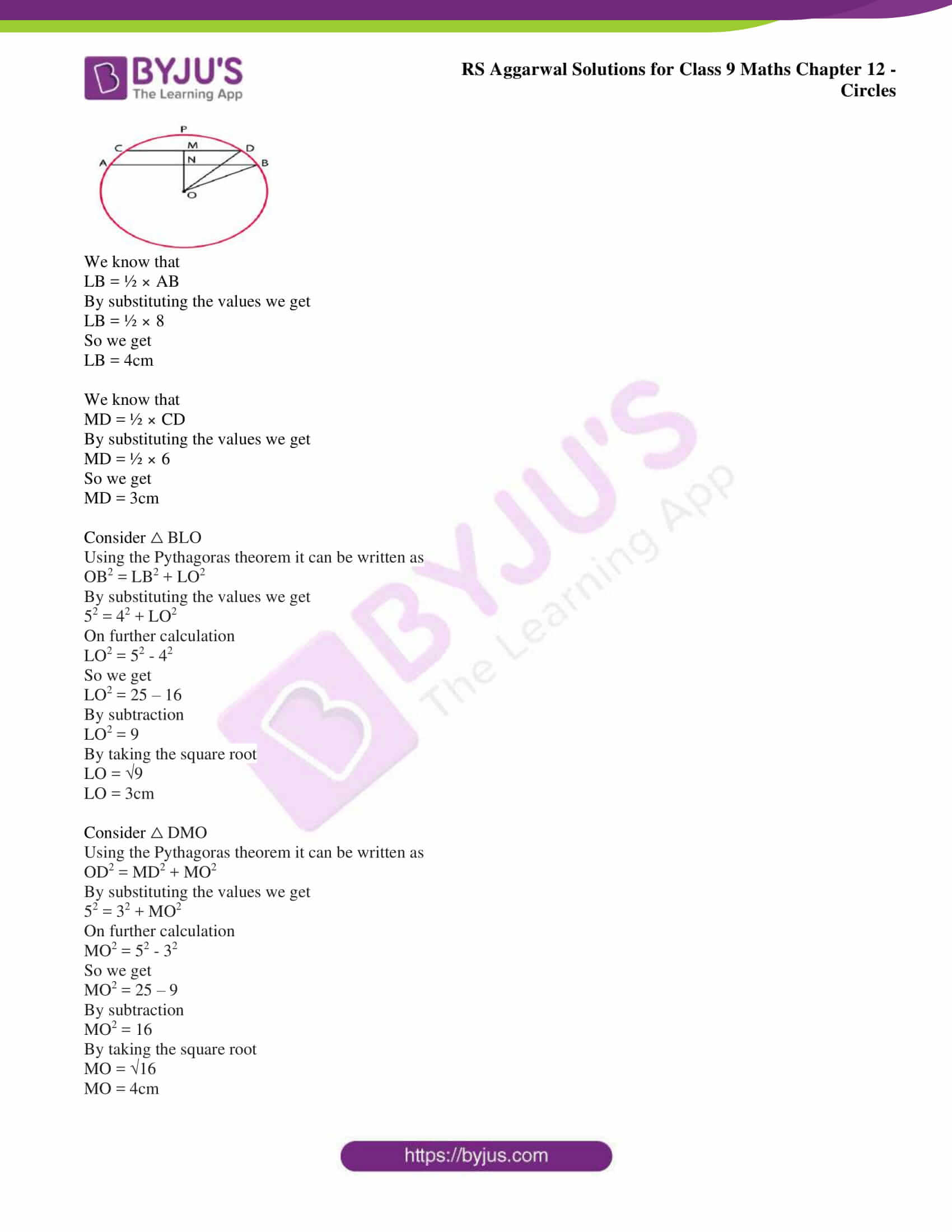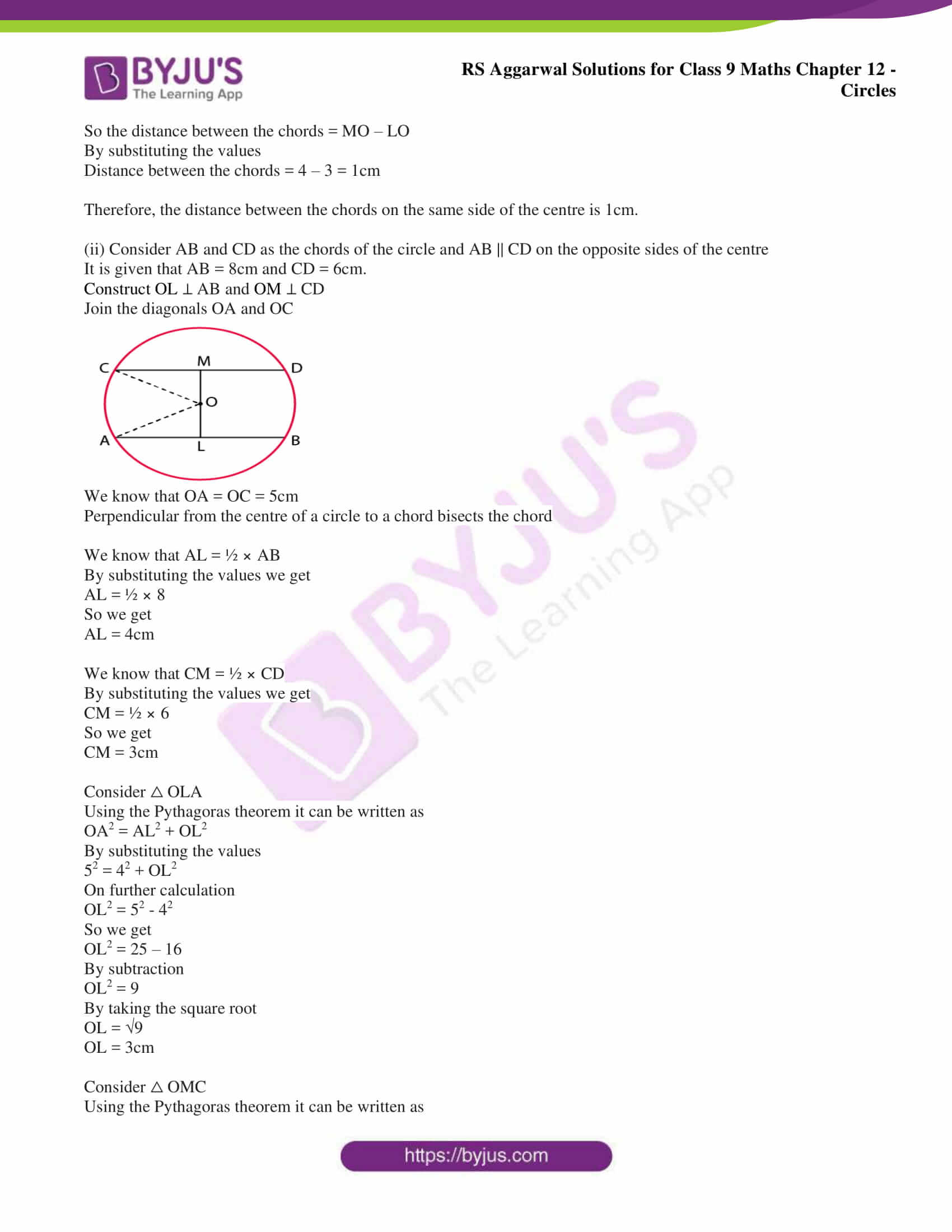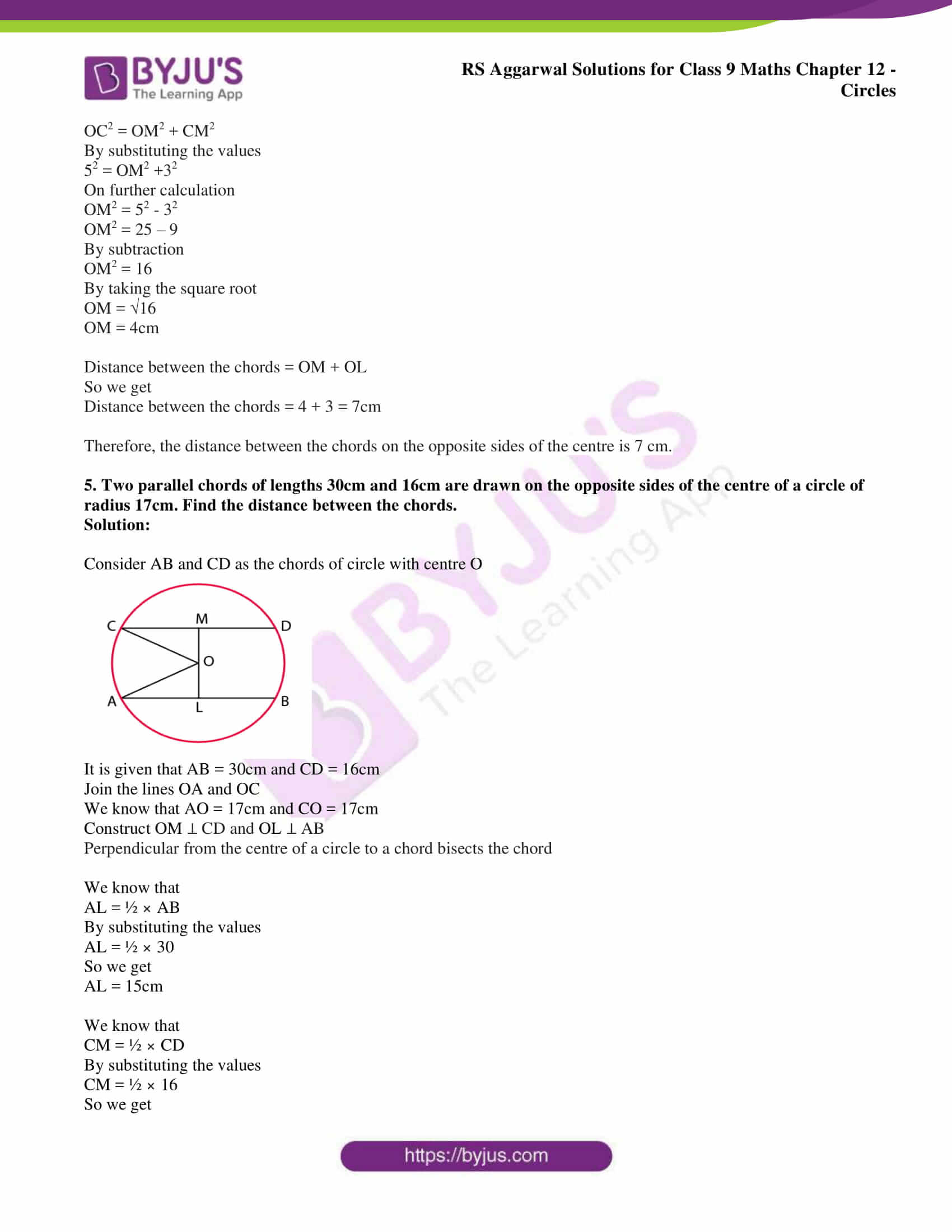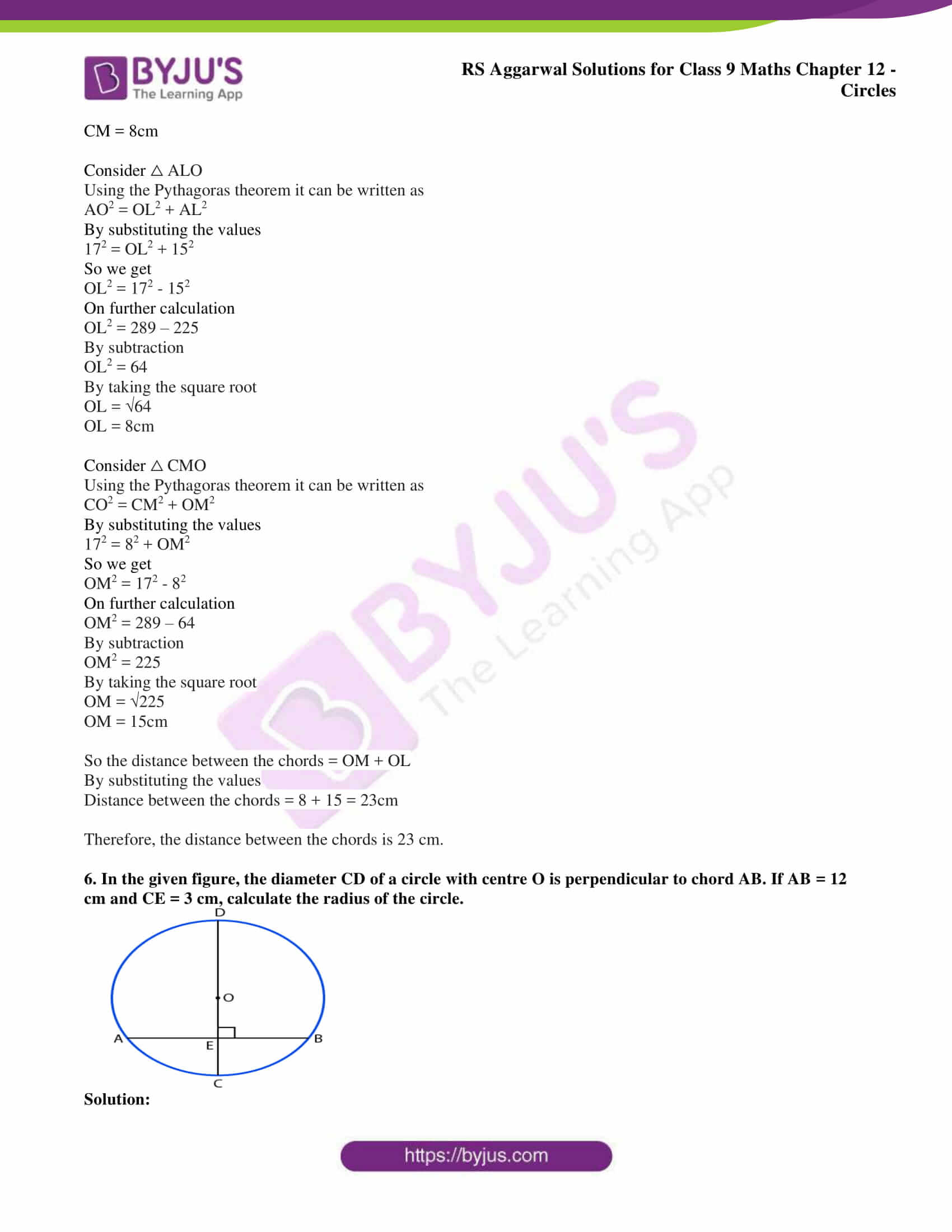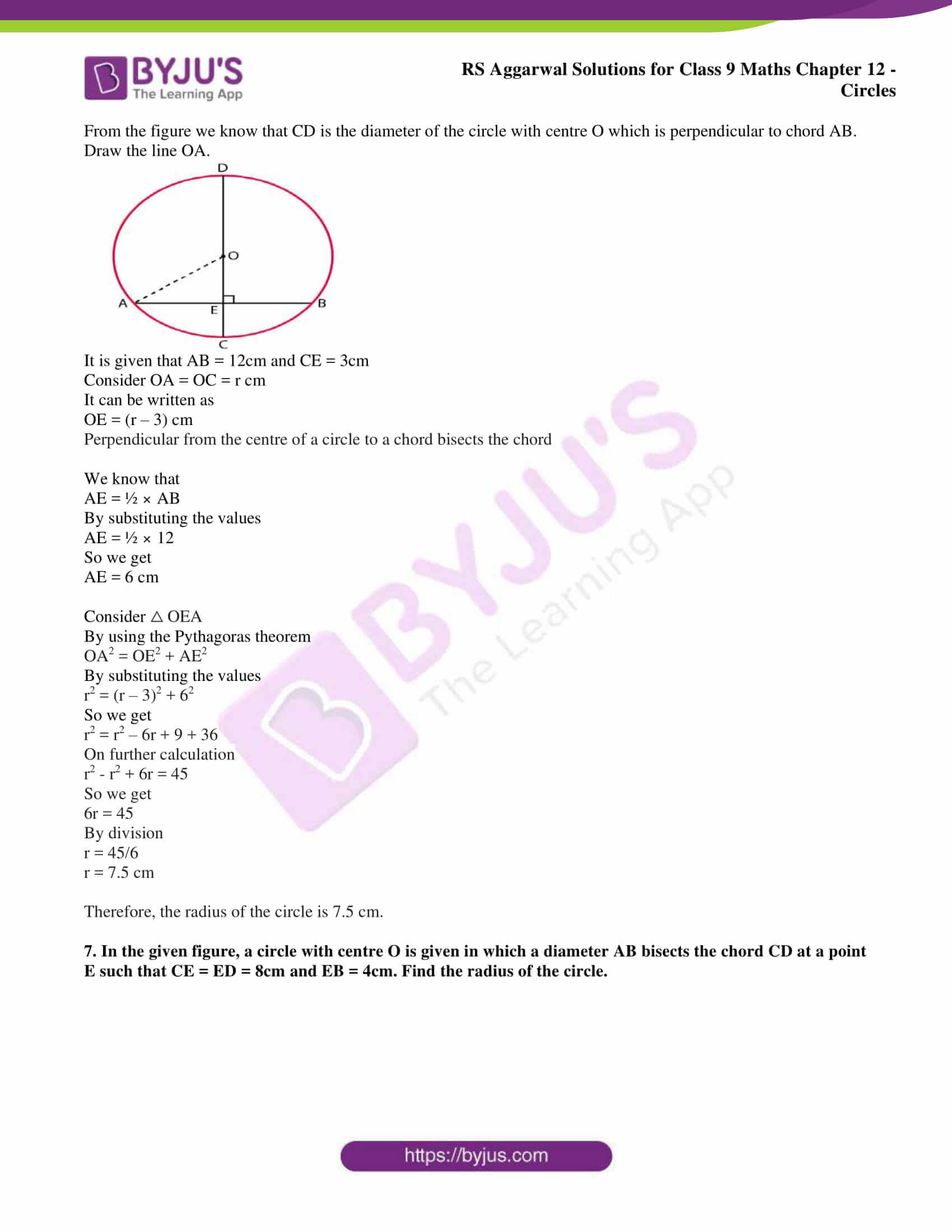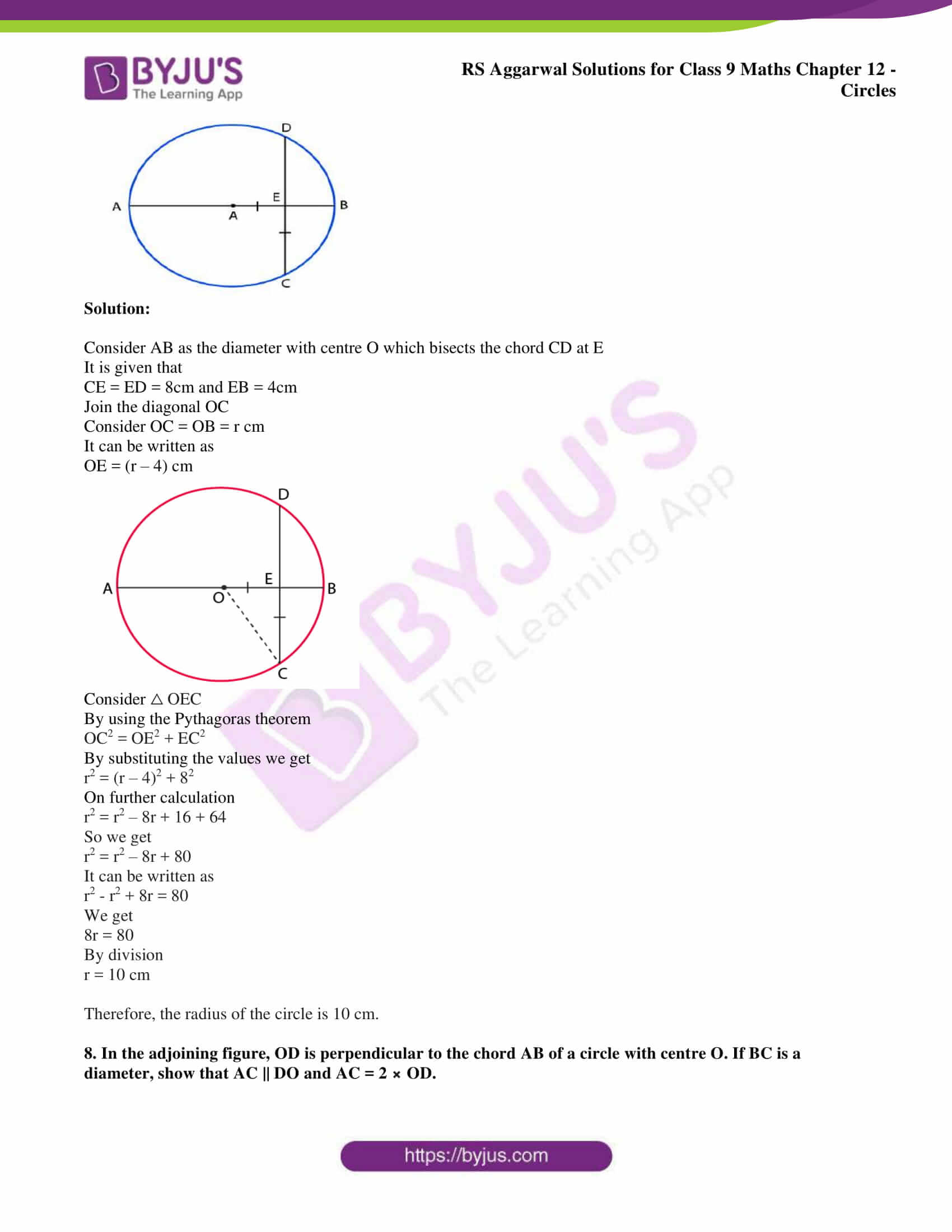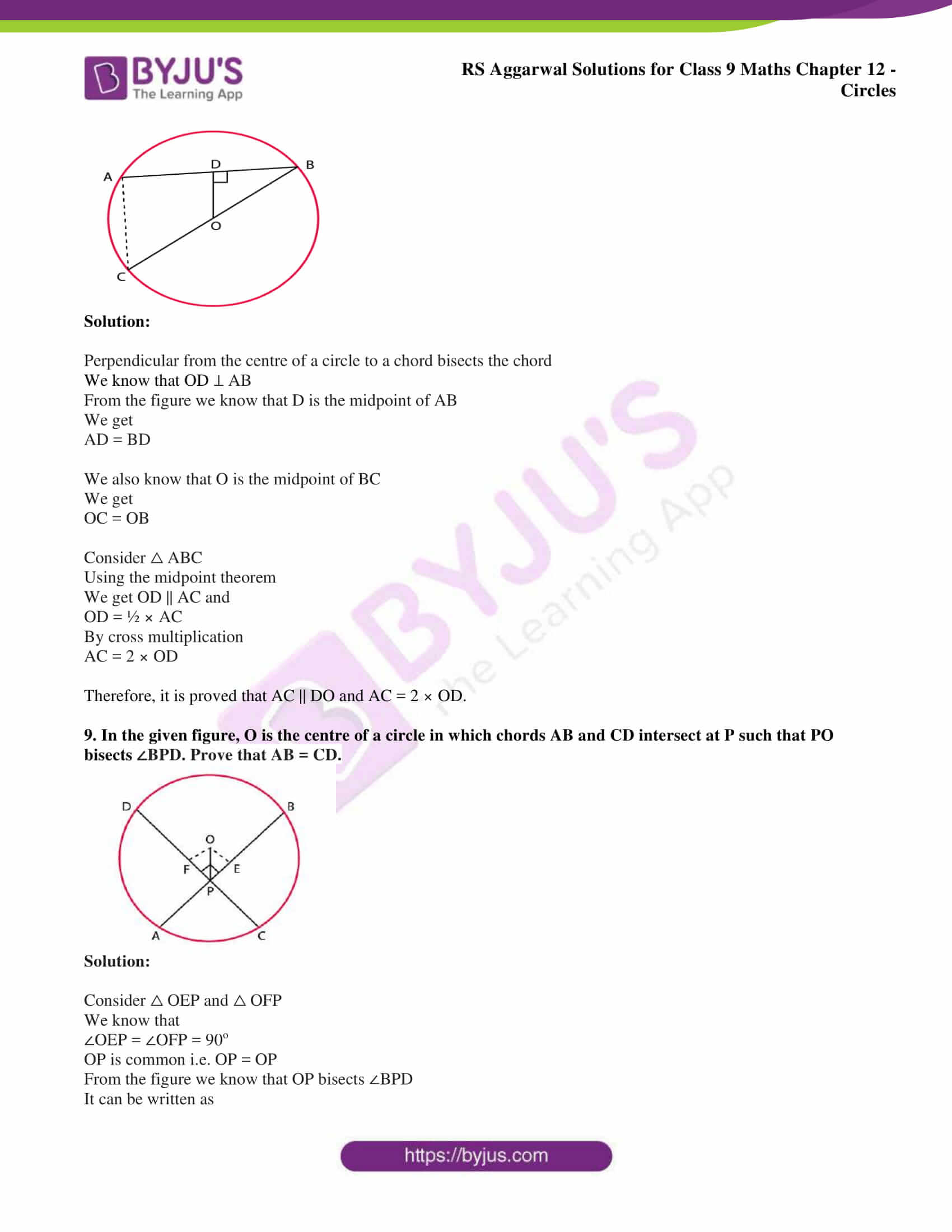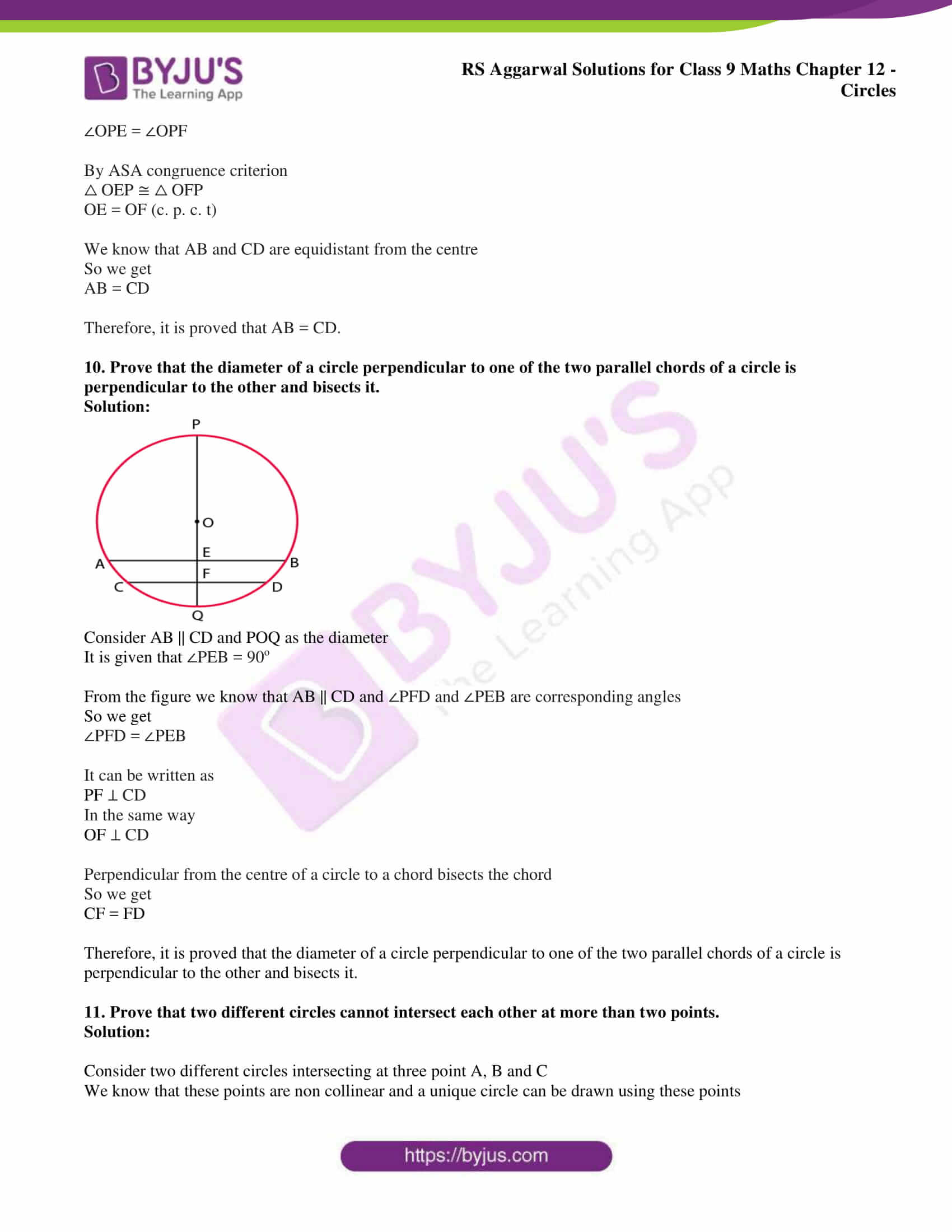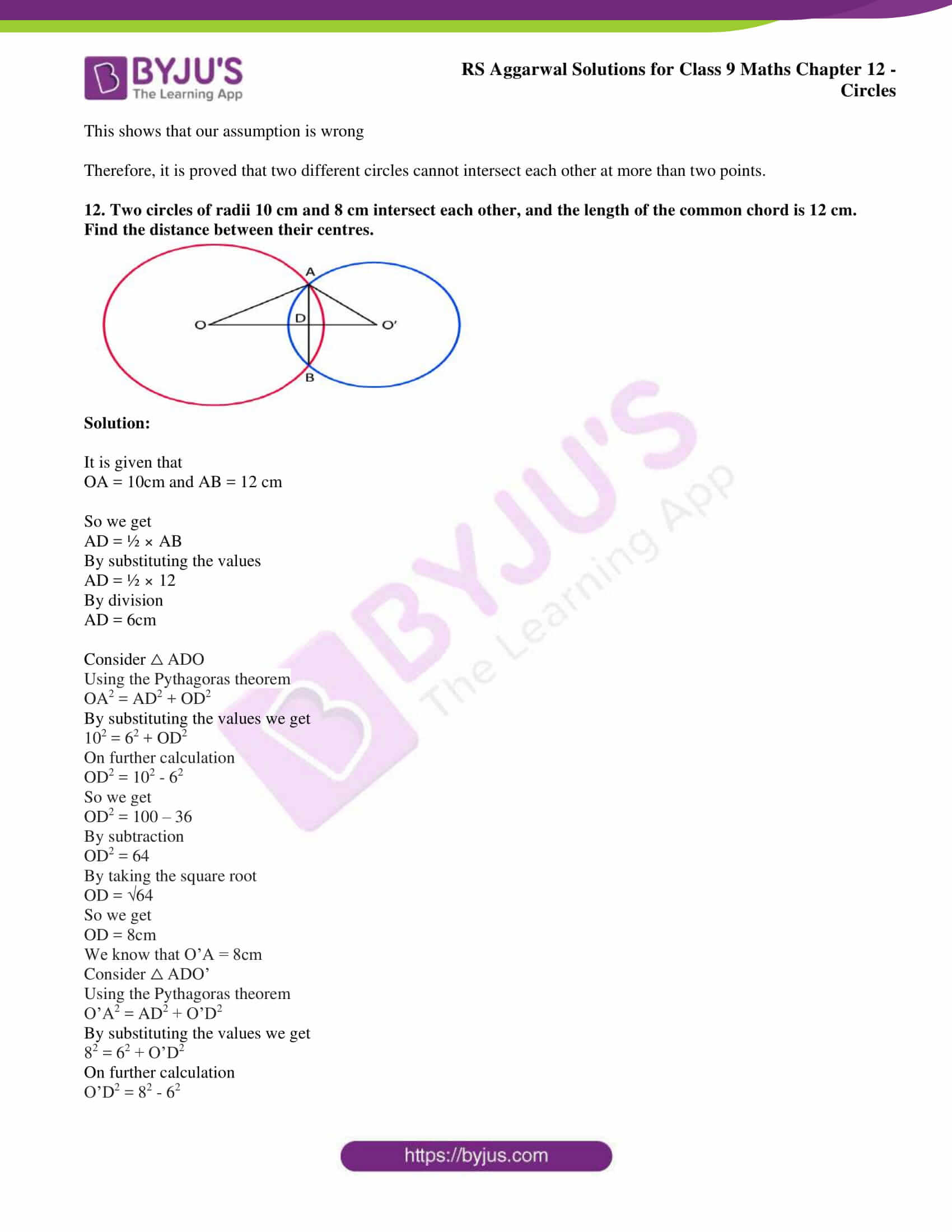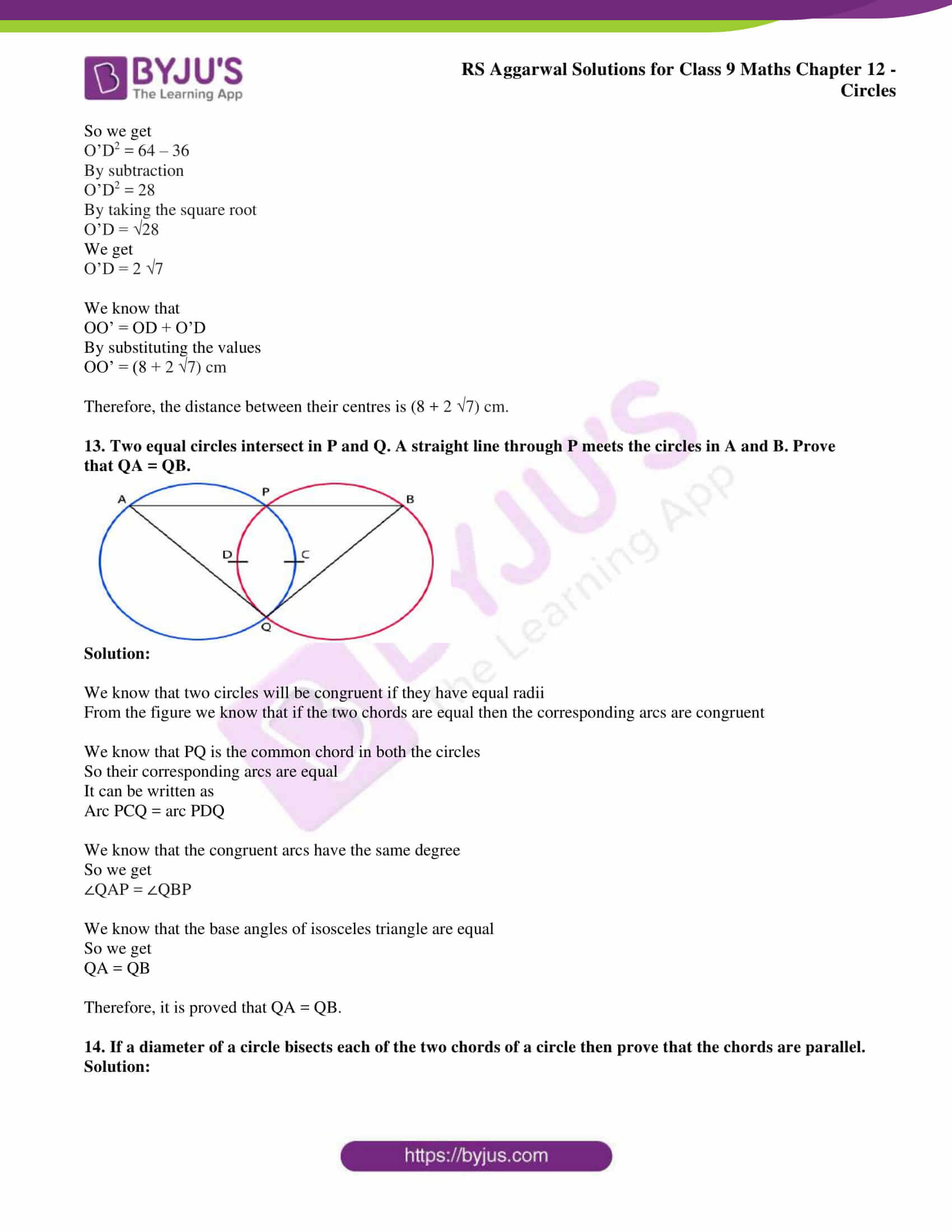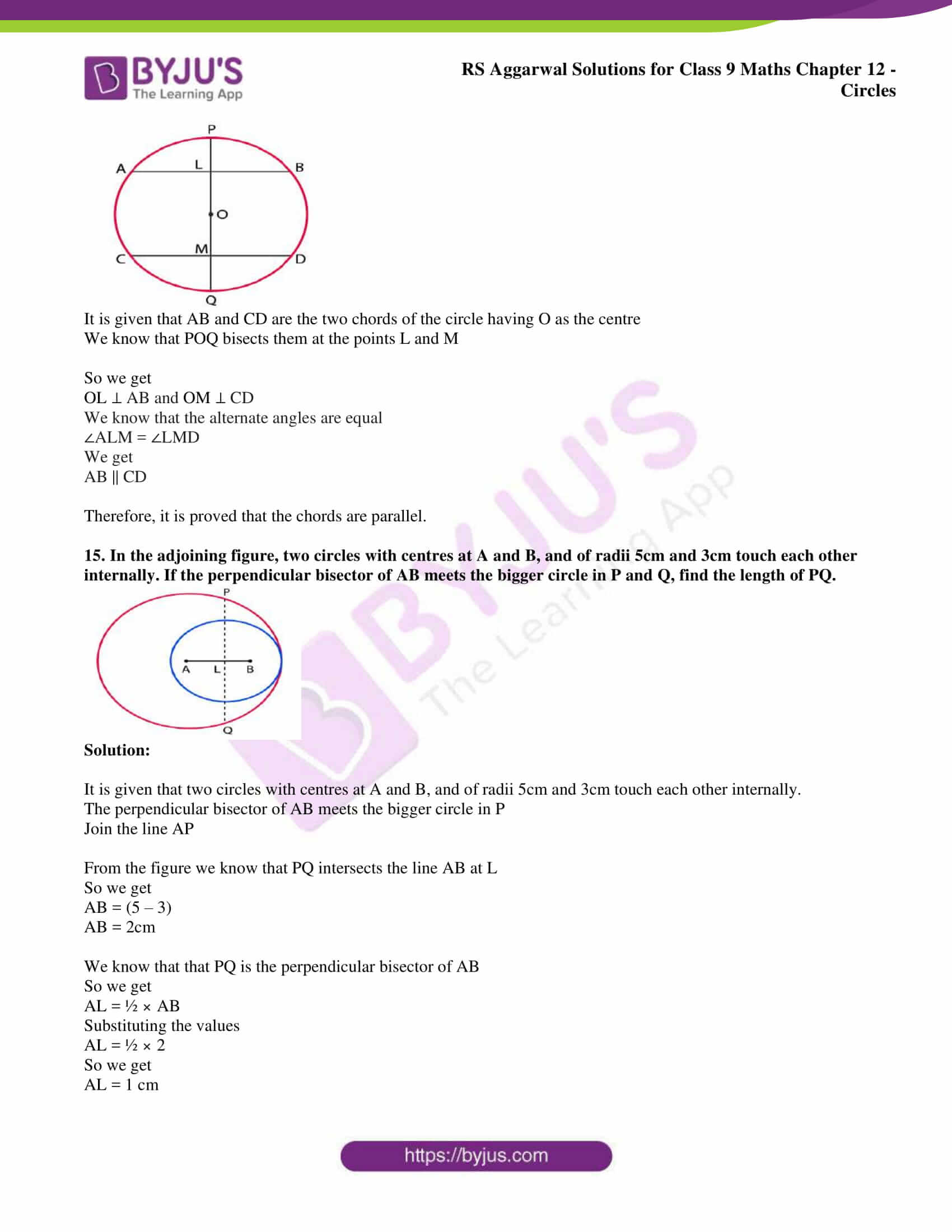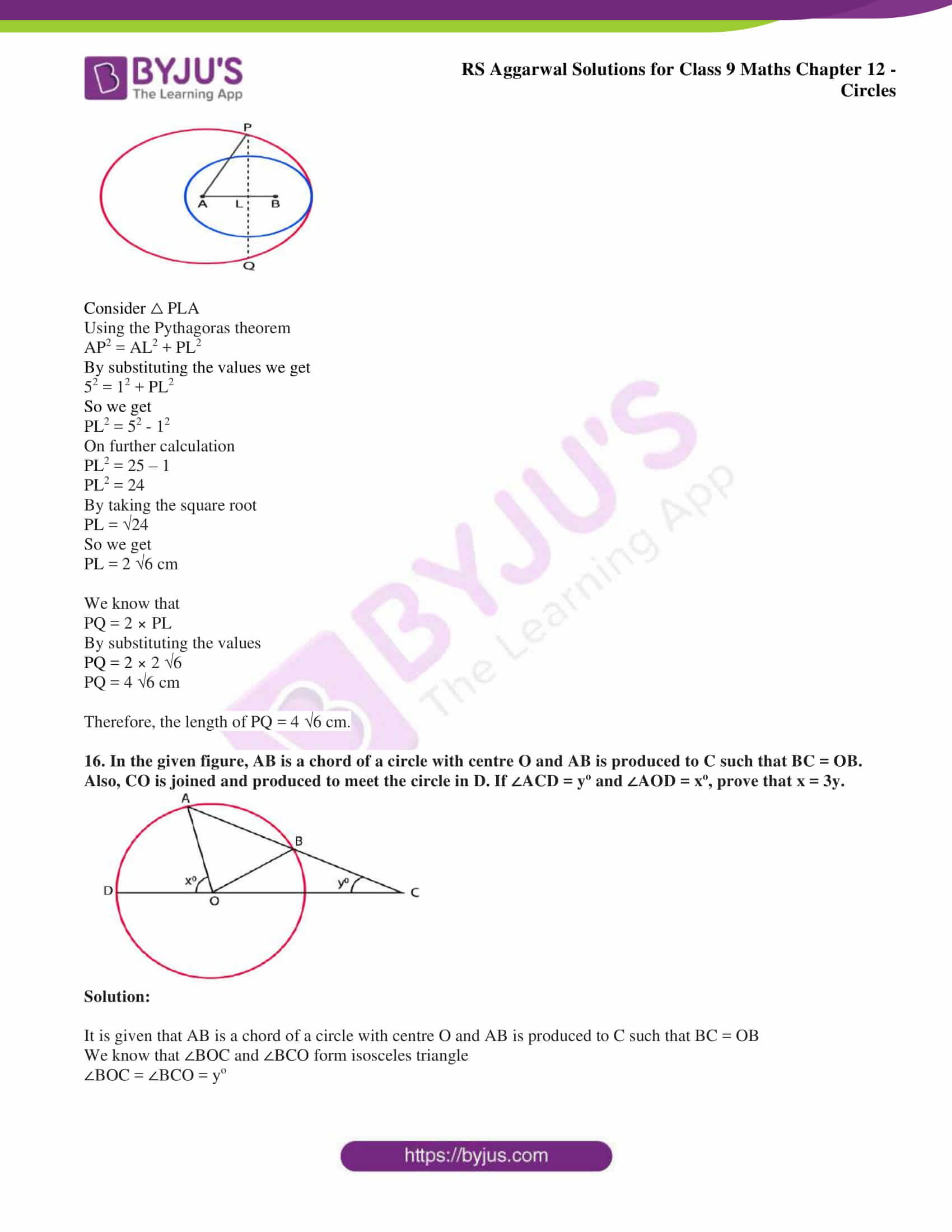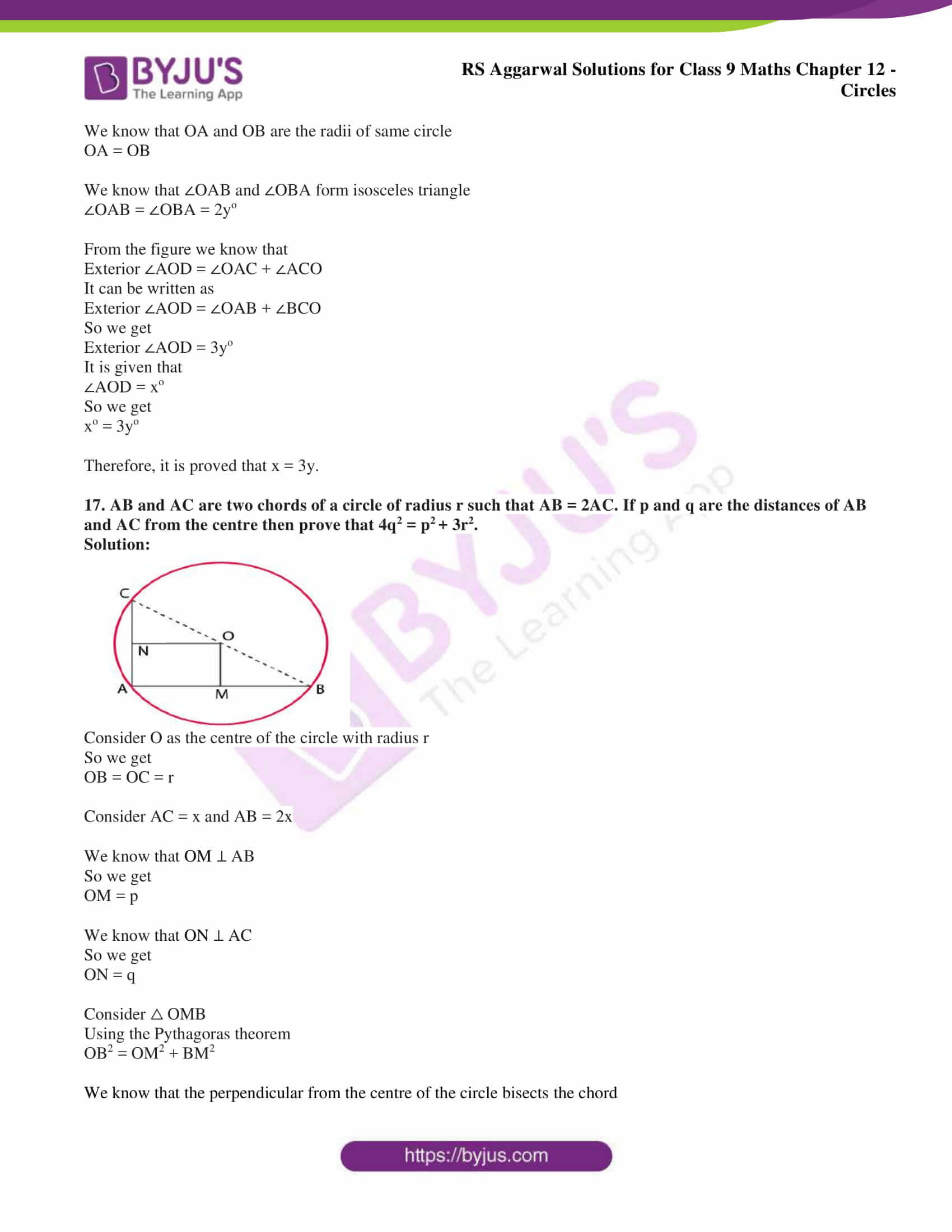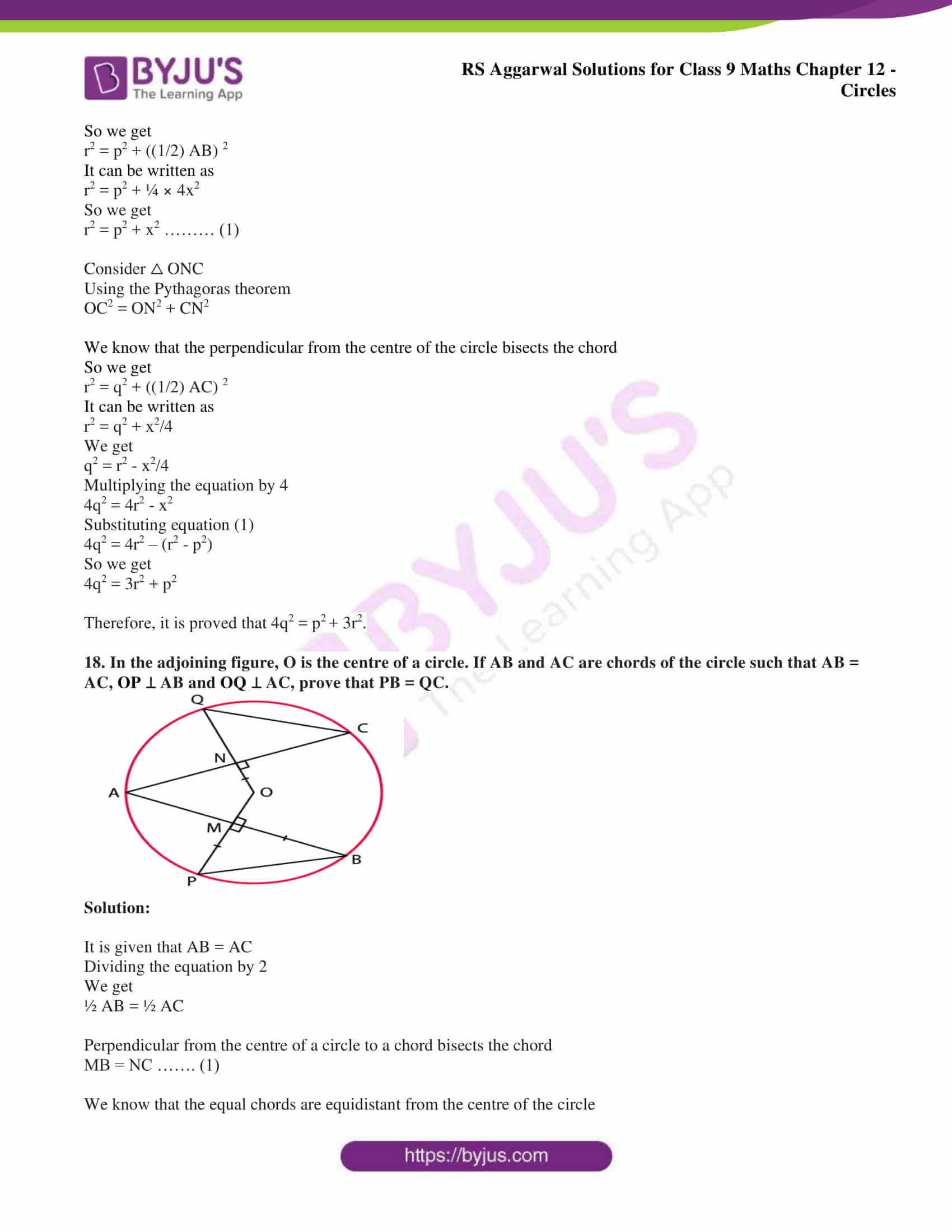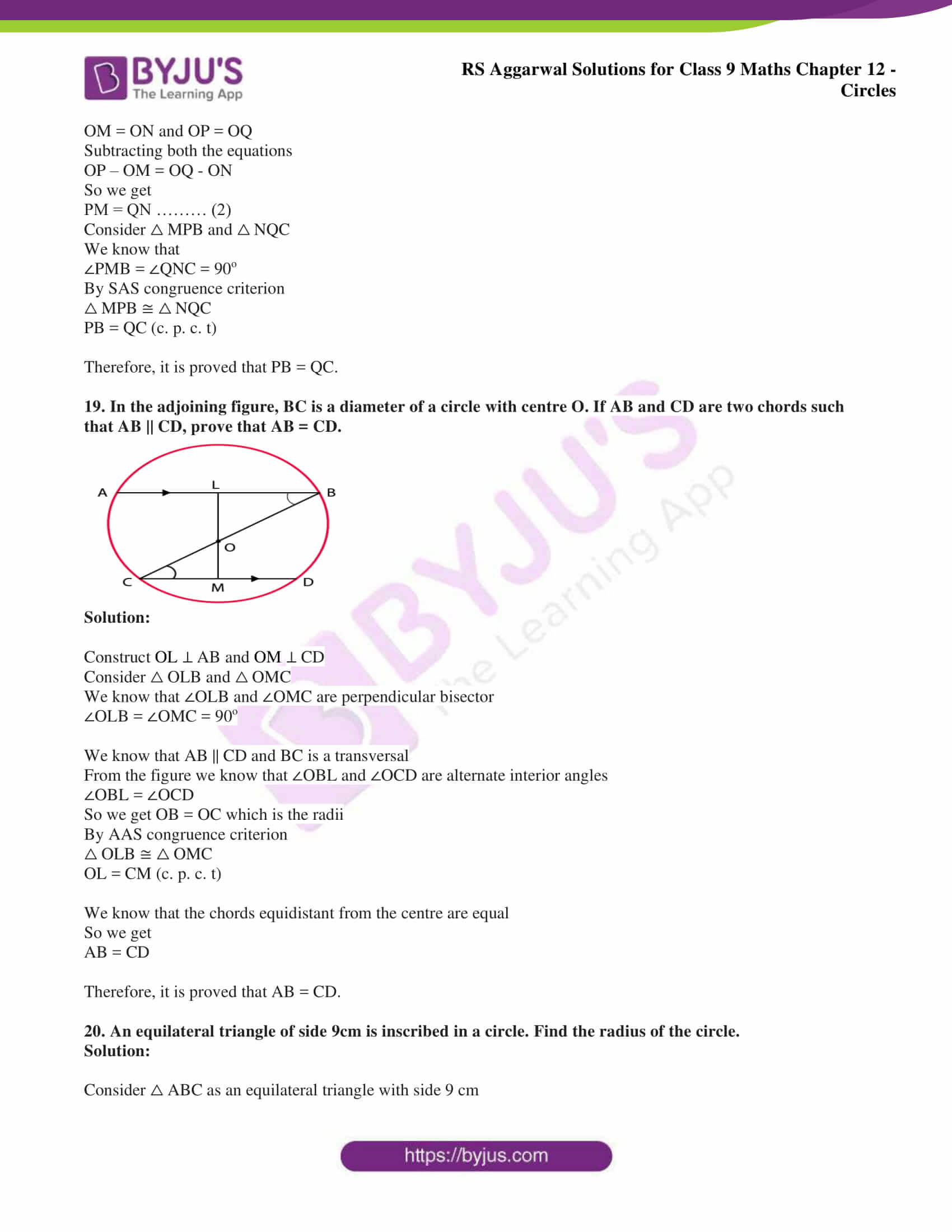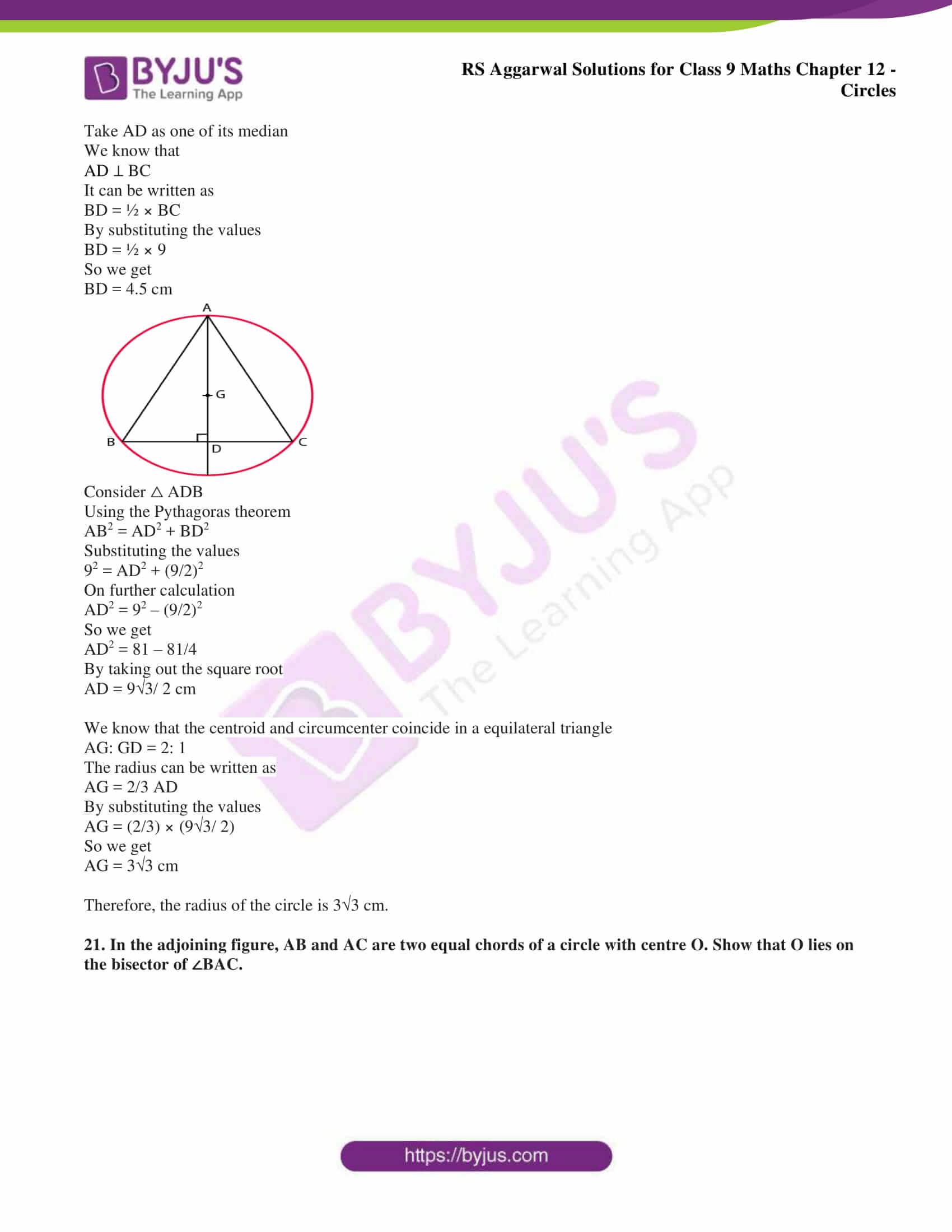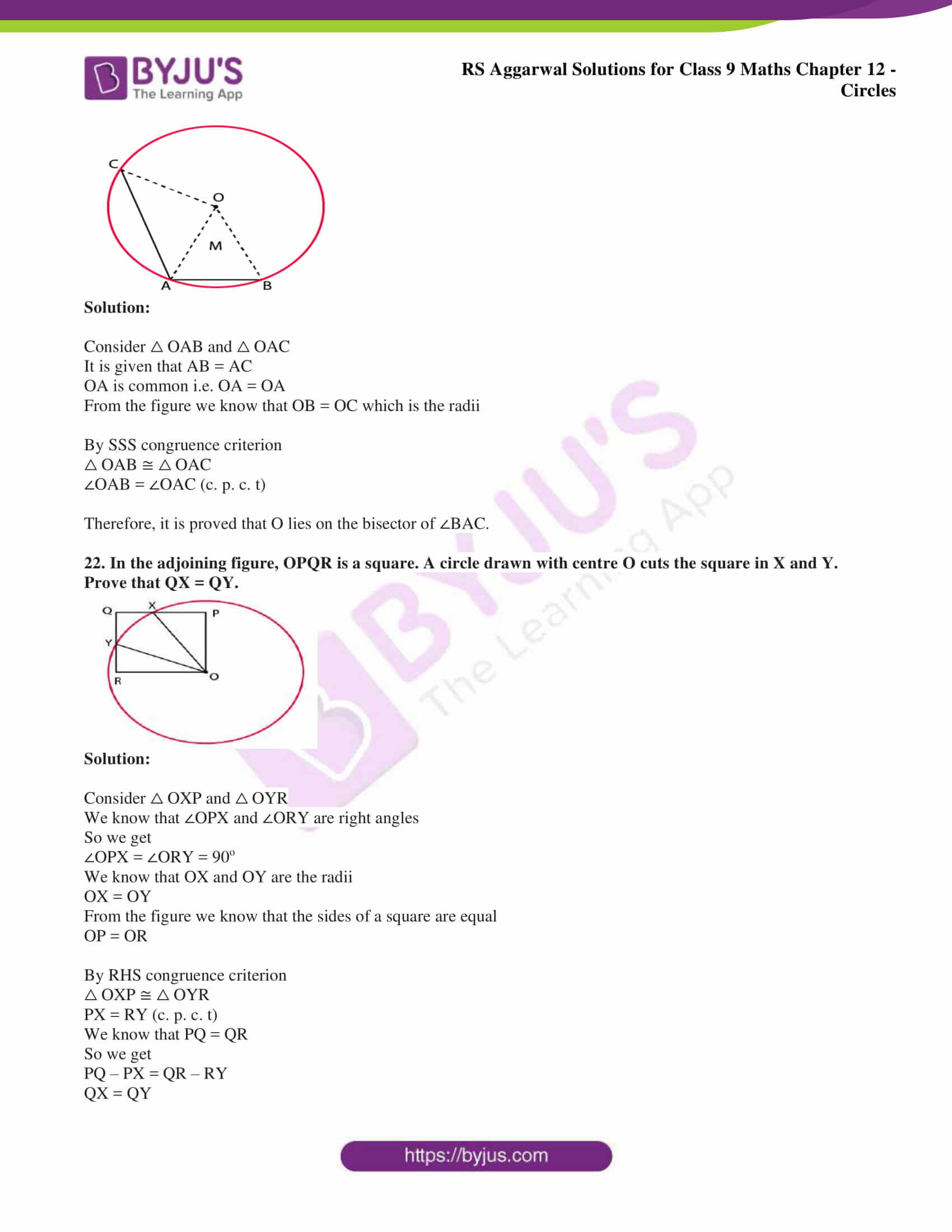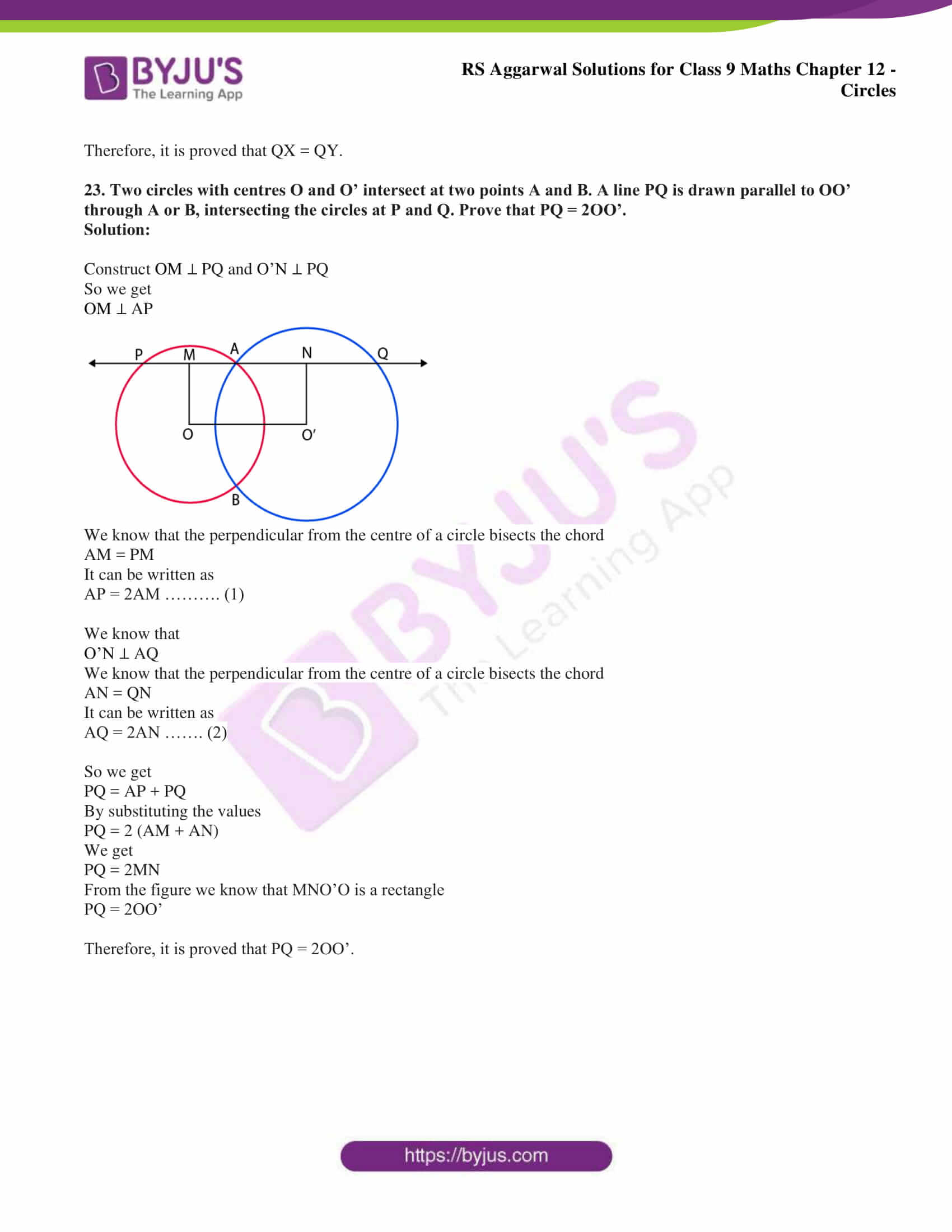## Exercise 12(A) page: 436

1. A chord of length 16cm is drawn in a circle of radius 10cm. Find the distance of the chord from the centre of the circle.

Solution:

Consider AB as the chord with O as the centre and radius 10cm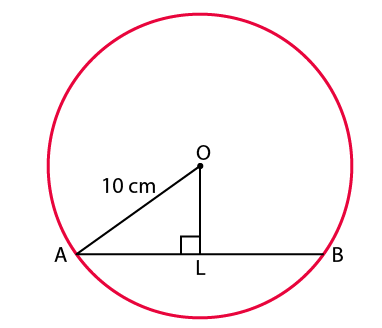So we get

OA = 10 cm and AB = 16cm

Construct OL ⊥ AB

Perpendicular from the centre of a circle to a chord bisects the chord

So we get

AL = ½ × AB

By substituting the values

AL = ½ × 16

So we get

AL = 8 cm

Consider △ OLA

Using the Pythagoras theorem it can be written as

OA2 = OL2 + AL2

By substituting the values we get

102 = OL2 + 82

On further calculation

OL2 = 102 – 82

So we get

OL2 = 100 – 64

By subtraction

OL2 = 36

By taking the square root

OL = √36

So we get

OL = 6cm

Therefore, the distance of the chord from the centre of the circle is 6cm.

2. Find the length of a chord which is at a distance of 3cm from the centre of a circle of radius 5cm.

Solution:

Consider AB as the chord of the circle with O as the centre and radius 5cm.

Construct OL ⊥ AB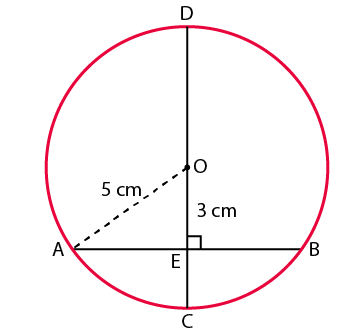It is given that OA = 5cm and OL = 3cm

Perpendicular from the centre of a circle to a chord bisects the chord

Consider △ OLA

Using the Pythagoras theorem it can be written as

OA2 = OL2 + AL2

By substituting the values we get

52 = OL2 + 32

On further calculation

OL2 = 52 – 32

So we get

OL2 = 25 – 9

By subtraction

OL2 = 16

By taking the square root

OL = √16

So we get

OL = 4cm

We know that

AB = 2AL

By substituting the values

AB = 2 × 4

So we get

AB = 8cm

Therefore, length of the chord is 8cm.

3. A chord of length 30cm is drawn at a distance of 8cm from the centre of a circle. Find out the radius of the circle.

Solution:

Consider AB as as the chord of the circle with O as the centre

Construct OL ⊥ AB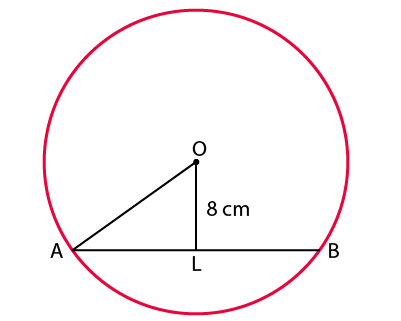From the figure we know that OL is the distance from the centre of chord

It is given that AB = 30cm and OL = 8cm

Perpendicular from the centre of a circle to a chord bisects the chord

So we get

AL = ½ × AB

By substituting the values

AL = ½ × 30

By division

AL = 15cm

Consider △ OLA

Using the Pythagoras theorem it can be written as

OA2 = OL2 + AL2

By substituting the values we get

OA2 = 82 + 152

On further calculation

OA2 = 64 + 225

OA2 = 289

By taking the square root

OA = √289

So we get

OA = 17cm

Therefore, the radius of the circle is 17cm.

4. In a circle of radius 5cm, AB and CD are two parallel chords of lengths 8cm and 6cm respectively. Calculate the distance between the chords if they are

(i) on the same side of the centre,

(ii) on the opposite sides of the centre.

Solution:

(i) Consider AB and CD as the two chords of the circle where AB || CD lying on the same side of the circle

It is given that AB = 8cm and OB = OD = 5cm

Draw a line to join the points OL and LM

Perpendicular from the centre of a circle to a chord bisects the chord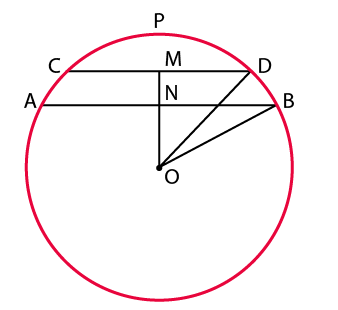We know that

LB = ½ × AB

By substituting the values we get

LB = ½ × 8

So we get

LB = 4cm

We know that

MD = ½ × CD

By substituting the values we get

MD = ½ × 6

So we get

MD = 3cm

Consider △ BLO

Using the Pythagoras theorem it can be written as

OB2 = LB2 + LO2

By substituting the values we get

52 = 42 + LO2

On further calculation

LO2 = 52 – 42

So we get

LO2 = 25 – 16

By subtraction

LO2 = 9

By taking the square root

LO = √9

LO = 3cm

Consider △ DMO

Using the Pythagoras theorem it can be written as

OD2 = MD2 + MO2

By substituting the values we get

52 = 32 + MO2

On further calculation

MO2 = 52 – 32

So we get

MO2 = 25 – 9

By subtraction

MO2 = 16

By taking the square root

MO = √16

MO = 4cm

So the distance between the chords = MO – LO

By substituting the values

Distance between the chords = 4 – 3 = 1cm

Therefore, the distance between the chords on the same side of the centre is 1cm.

(ii) Consider AB and CD as the chords of the circle and AB || CD on the opposite sides of the centre

It is given that AB = 8cm and CD = 6cm.

Construct OL ⊥ AB and OM ⊥ CD

Join the diagonals OA and OC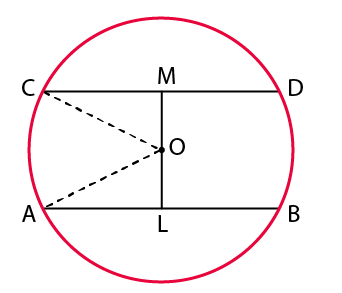We know that OA = OC = 5cm

Perpendicular from the centre of a circle to a chord bisects the chord

We know that AL = ½ × AB

By substituting the values we get

AL = ½ × 8

So we get

AL = 4cm

We know that CM = ½ × CD

By substituting the values we get

CM = ½ × 6

So we get

CM = 3cm

Consider △ OLA

Using the Pythagoras theorem it can be written as

OA2 = AL2 + OL2

By substituting the values

52 = 42 + OL2

On further calculation

OL2 = 52 – 42

So we get

OL2 = 25 – 16

By subtraction

OL2 = 9

By taking the square root

OL = √9

OL = 3cm

Consider △ OMC

Using the Pythagoras theorem it can be written as

OC2 = OM2 + CM2

By substituting the values

52 = OM2 +32

On further calculation

OM2 = 52 – 32

OM2 = 25 – 9

By subtraction

OM2 = 16

By taking the square root

OM = √16

OM = 4cm

Distance between the chords = OM + OL

So we get

Distance between the chords = 4 + 3 = 7cm

Therefore, the distance between the chords on the opposite sides of the centre is 7 cm.

5. Two parallel chords of lengths 30cm and 16cm are drawn on the opposite sides of the centre of a circle of radius 17cm. Find the distance between the chords.

Solution:

Consider AB and CD as the chords of circle with centre O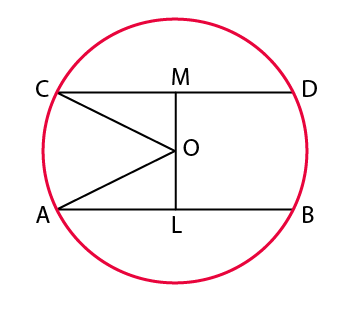It is given that AB = 30cm and CD = 16cm

Join the lines OA and OC

We know that AO = 17cm and CO = 17cm

Construct OM ⊥ CD and OL ⊥ AB

Perpendicular from the centre of a circle to a chord bisects the chord

We know that

AL = ½ × AB

By substituting the values

AL = ½ × 30

So we get

AL = 15cm

We know that

CM = ½ × CD

By substituting the values

CM = ½ × 16

So we get

CM = 8cm

Consider △ ALO

Using the Pythagoras theorem it can be written as

AO2 = OL2 + AL2

By substituting the values

172 = OL2 + 152

So we get

OL2 = 172 – 152

On further calculation

OL2 = 289 – 225

By subtraction

OL2 = 64

By taking the square root

OL = √64

OL = 8cm

Consider △ CMO

Using the Pythagoras theorem it can be written as

CO2 = CM2 + OM2

By substituting the values

172 = 82 + OM2

So we get

OM2 = 172 – 82

On further calculation

OM2 = 289 – 64

By subtraction

OM2 = 225

By taking the square root

OM = √225

OM = 15cm

So the distance between the chords = OM + OL

By substituting the values

Distance between the chords = 8 + 15 = 23cm

Therefore, the distance between the chords is 23 cm.

6. In the given figure, the diameter CD of a circle with centre O is perpendicular to chord AB. If AB = 12 cm and CE = 3 cm, calculate the radius of the circle.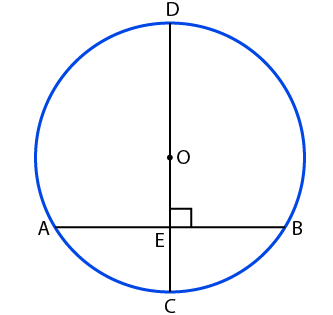Solution:

From the figure we know that CD is the diameter of the circle with centre O which is perpendicular to chord AB.

Draw the line OA.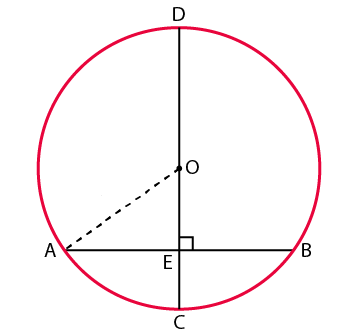It is given that AB = 12cm and CE = 3cm

Consider OA = OC = r cm

It can be written as

OE = (r – 3) cm

Perpendicular from the centre of a circle to a chord bisects the chord

We know that

AE = ½ × AB

By substituting the values

AE = ½ × 12

So we get

AE = 6 cm

Consider △ OEA

By using the Pythagoras theorem

OA2 = OE2 + AE2

By substituting the values

r2 = (r – 3)2 + 62

So we get

r2 = r2 – 6r + 9 + 36

On further calculation

r2 – r2 + 6r = 45

So we get

6r = 45

By division

r = 45/6

r = 7.5 cm

Therefore, the radius of the circle is 7.5 cm.

7. In the given figure, a circle with centre O is given in which a diameter AB bisects the chord CD at a point E such that CE = ED = 8cm and EB = 4cm. Find the radius of the circle.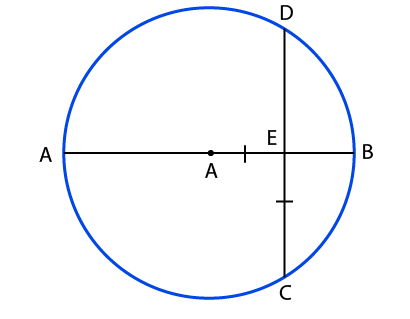Solution:

Consider AB as the diameter with centre O which bisects the chord CD at E

It is given that

CE = ED = 8cm and EB = 4cm

Join the diagonal OC

Consider OC = OB = r cm

It can be written as

OE = (r – 4) cm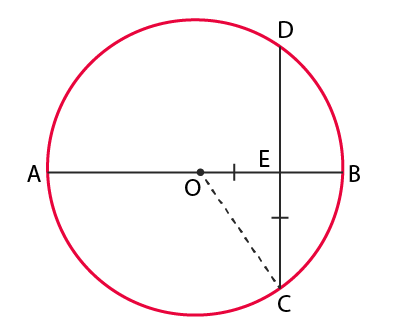Consider △ OEC

By using the Pythagoras theorem

OC2 = OE2 + EC2

By substituting the values we get

r2 = (r – 4)2 + 82

On further calculation

r2 = r2 – 8r + 16 + 64

So we get

r2 = r2 – 8r + 80

It can be written as

r2 – r2 + 8r = 80

We get

8r = 80

By division

r = 10 cm

Therefore, the radius of the circle is 10 cm.

8. In the adjoining figure, OD is perpendicular to the chord AB of a circle with centre O. If BC is a diameter, show that AC || DO and AC = 2 × OD.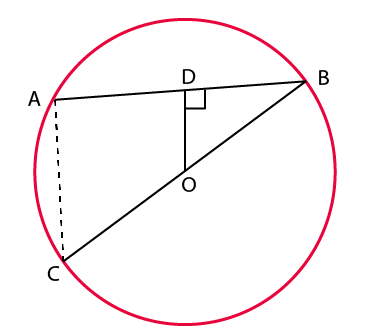Solution:

Perpendicular from the centre of a circle to a chord bisects the chord

We know that OD ⊥ AB

From the figure we know that D is the midpoint of AB

We get

We also know that O is the midpoint of BC

We get

OC = OB

Consider △ ABC

Using the midpoint theorem

We get OD || AC and

OD = ½ × AC

By cross multiplication

AC = 2 × OD

Therefore, it is proved that AC || DO and AC = 2 × OD.

9. In the given figure, O is the centre of a circle in which chords AB and CD intersect at P such that PO bisects ∠BPD. Prove that AB = CD.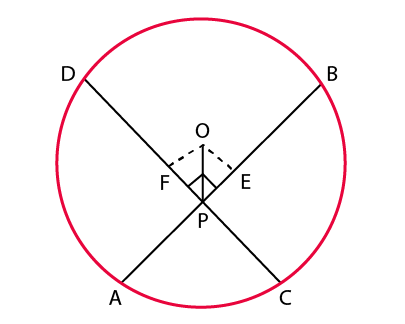Solution:

Consider △ OEP and △ OFP

We know that

∠OEP = ∠OFP = 90o

OP is common i.e. OP = OP

From the figure we know that OP bisects ∠BPD

It can be written as

∠OPE = ∠OPF

By ASA congruence criterion

△ OEP ≅ △ OFP

OE = OF (c. p. c. t)

We know that AB and CD are equidistant from the centre

So we get

AB = CD

Therefore, it is proved that AB = CD.

10. Prove that the diameter of a circle perpendicular to one of the two parallel chords of a circle is perpendicular to the other and bisects it.

Solution: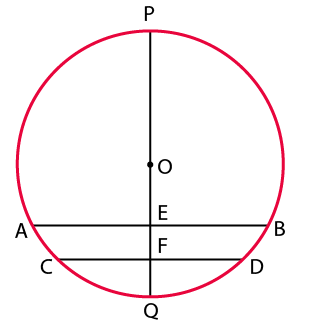Consider AB || CD and POQ as the diameter

It is given that ∠PEB = 90o

From the figure we know that AB || CD and ∠PFD and ∠PEB are corresponding angles

So we get

∠PFD = ∠PEB

It can be written as

PF ⊥ CD

In the same way

OF ⊥ CD

Perpendicular from the centre of a circle to a chord bisects the chord

So we get

CF = FD

Therefore, it is proved that the diameter of a circle perpendicular to one of the two parallel chords of a circle is perpendicular to the other and bisects it.

11. Prove that two different circles cannot intersect each other at more than two points.

Solution:

Consider two different circles intersecting at three point A, B and C

We know that these points are non collinear and a unique circle can be drawn using these points

This shows that our assumption is wrong

Therefore, it is proved that two different circles cannot intersect each other at more than two points.

12. Two circles of radii 10 cm and 8 cm intersect each other, and the length of the common chord is 12 cm. Find the distance between their centres.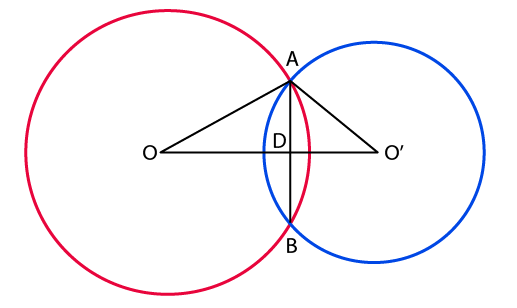Solution:

It is given that

OA = 10cm and AB = 12 cm

So we get

By substituting the values

By division

Using the Pythagoras theorem

By substituting the values we get

102 = 62 + OD2

On further calculation

OD2 = 102 – 62

So we get

OD2 = 100 – 36

By subtraction

OD2 = 64

By taking the square root

OD = √64

So we get

OD = 8cm

We know that O’A = 8cm

Using the Pythagoras theorem

By substituting the values we get

82 = 62 + O’D2

On further calculation

O’D2 = 82 – 62

So we get

O’D2 = 64 – 36

By subtraction

O’D2 = 28

By taking the square root

O’D = √28

We get

O’D = 2 √7

We know that

OO’ = OD + O’D

By substituting the values

OO’ = (8 + 2 √7) cm

Therefore, the distance between their centres is (8 + 2 √7) cm.

13. Two equal circles intersect in P and Q. A straight line through P meets the circles in A and B. Prove that QA = QB.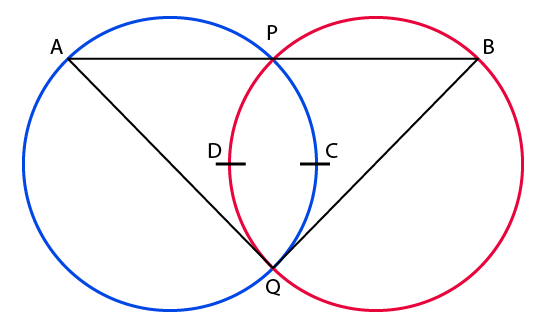Solution:

We know that two circles will be congruent if they have equal radii

From the figure we know that if the two chords are equal then the corresponding arcs are congruent

We know that PQ is the common chord in both the circles

So their corresponding arcs are equal

It can be written as

Arc PCQ = arc PDQ

We know that the congruent arcs have the same degree

So we get

∠QAP = ∠QBP

We know that the base angles of isosceles triangle are equal

So we get

QA = QB

Therefore, it is proved that QA = QB.

14. If a diameter of a circle bisects each of the two chords of a circle then prove that the chords are parallel.

Solution: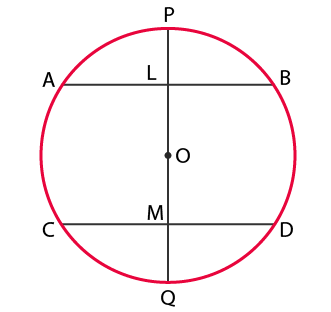It is given that AB and CD are the two chords of the circle having O as the centre

We know that POQ bisects them at the points L and M

So we get

OL ⊥ AB and OM ⊥ CD

We know that the alternate angles are equal

∠ALM = ∠LMD

We get

AB || CD

Therefore, it is proved that the chords are parallel.

15. In the adjoining figure, two circles with centres at A and B, and of radii 5cm and 3cm touch each other internally. If the perpendicular bisector of AB meets the bigger circle in P and Q, find the length of PQ.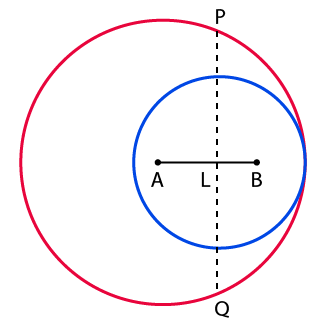Solution:

It is given that two circles with centres at A and B, and of radii 5cm and 3cm touch each other internally.

The perpendicular bisector of AB meets the bigger circle in P

Join the line AP

From the figure we know that PQ intersects the line AB at L

So we get

AB = (5 – 3)

AB = 2cm

We know that that PQ is the perpendicular bisector of AB

So we get

AL = ½ × AB

Substituting the values

AL = ½ × 2

So we get

AL = 1 cm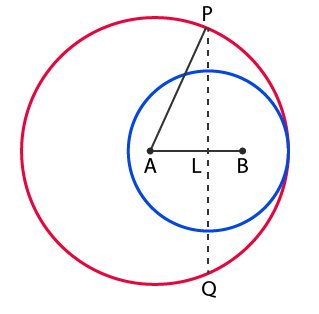Consider △ PLA

Using the Pythagoras theorem

AP2 = AL2 + PL2

By substituting the values we get

52 = 12 + PL2

So we get

PL2 = 52 – 12

On further calculation

PL2 = 25 – 1

PL2 = 24

By taking the square root

PL = √24

So we get

PL = 2 √6 cm

We know that

PQ = 2 × PL

By substituting the values

PQ = 2 × 2 √6

PQ = 4 √6 cm

Therefore, the length of PQ = 4 √6 cm.

16. In the given figure, AB is a chord of a circle with centre O and AB is produced to C such that BC = OB. Also, CO is joined and produced to meet the circle in D. If ∠ACD = yo and ∠AOD = xo, prove that x = 3y.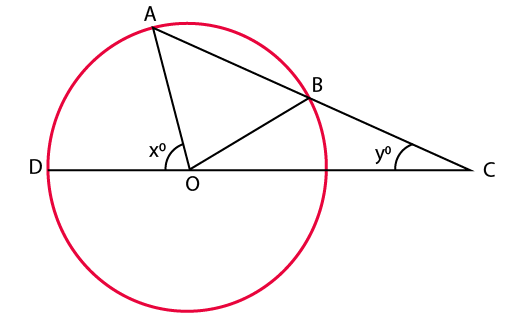Solution:

It is given that AB is a chord of a circle with centre O and AB is produced to C such that BC = OB

We know that ∠BOC and ∠BCO form isosceles triangle

∠BOC = ∠BCO = yo

We know that OA and OB are the radii of same circle

OA = OB

We know that ∠OAB and ∠OBA form isosceles triangle

∠OAB = ∠OBA = 2yo

From the figure we know that

Exterior ∠AOD = ∠OAC + ∠ACO

It can be written as

Exterior ∠AOD = ∠OAB + ∠BCO

So we get

Exterior ∠AOD = 3yo

It is given that

∠AOD = xo

So we get

xo = 3yo

Therefore, it is proved that x = 3y.

17. AB and AC are two chords of a circle of radius r such that AB = 2AC. If p and q are the distances of AB and AC from the centre then prove that 4q2 = p2 + 3r2.

Solution: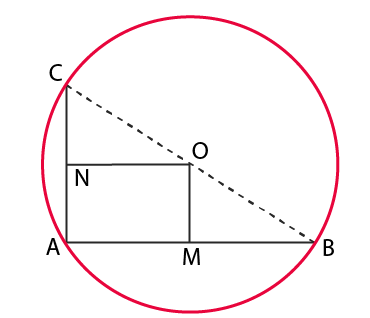Consider O as the centre of the circle with radius r

So we get

OB = OC = r

Consider AC = x and AB = 2x

We know that OM ⊥ AB

So we get

OM = p

We know that ON ⊥ AC

So we get

ON = q

Consider △ OMB

Using the Pythagoras theorem

OB2 = OM2 + BM2

We know that the perpendicular from the centre of the circle bisects the chord

So we get

r2 = p2 + ((1/2) AB) 2

It can be written as

r2 = p2 + ¼ × 4x2

So we get

r2 = p2 + x2 ……… (1)

Consider △ ONC

Using the Pythagoras theorem

OC2 = ON2 + CN2

We know that the perpendicular from the centre of the circle bisects the chord

So we get

r2 = q2 + ((1/2) AC) 2

It can be written as

r2 = q2 + x2/4

We get

q2 = r2 – x2/4

Multiplying the equation by 4

4q2 = 4r2 – x2

Substituting equation (1)

4q2 = 4r2 – (r2 – p2)

So we get

4q2 = 3r2 + p2

Therefore, it is proved that 4q2 = p2 + 3r2.

18. In the adjoining figure, O is the centre of a circle. If AB and AC are chords of the circle such that AB = AC, OP ⊥ AB and OQ ⊥ AC, prove that PB = QC.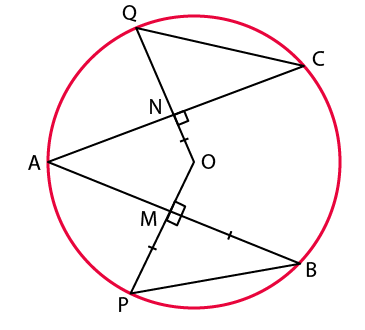Solution:

It is given that AB = AC

Dividing the equation by 2

We get

½ AB = ½ AC

Perpendicular from the centre of a circle to a chord bisects the chord

MB = NC ……. (1)

We know that the equal chords are equidistant from the centre of the circle

OM = ON and OP = OQ

Subtracting both the equations

OP – OM = OQ – ON

So we get

PM = QN ……… (2)

Consider △ MPB and △ NQC

We know that

∠PMB = ∠QNC = 90o

By SAS congruence criterion

△ MPB ≅ △ NQC

PB = QC (c. p. c. t)

Therefore, it is proved that PB = QC.

19. In the adjoining figure, BC is a diameter of a circle with centre O. If AB and CD are two chords such that AB || CD, prove that AB = CD.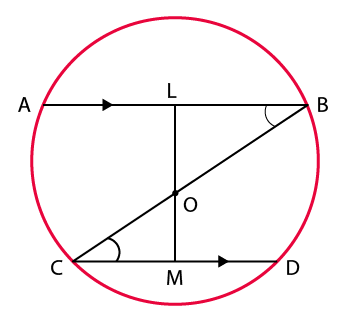Solution:

Construct OL ⊥ AB and OM ⊥ CD

Consider △ OLB and △ OMC

We know that ∠OLB and ∠OMC are perpendicular bisector

∠OLB = ∠OMC = 90o

We know that AB || CD and BC is a transversal

From the figure we know that ∠OBL and ∠OCD are alternate interior angles

∠OBL = ∠OCD

So we get OB = OC which is the radii

By AAS congruence criterion

△ OLB ≅ △ OMC

OL = CM (c. p. c. t)

We know that the chords equidistant from the centre are equal

So we get

AB = CD

Therefore, it is proved that AB = CD.

20. An equilateral triangle of side 9cm is inscribed in a circle. Find the radius of the circle.

Solution:

Consider △ ABC as an equilateral triangle with side 9 cm

Take AD as one of its median

We know that

It can be written as

BD = ½ × BC

By substituting the values

BD = ½ × 9

So we get

BD = 4.5 cm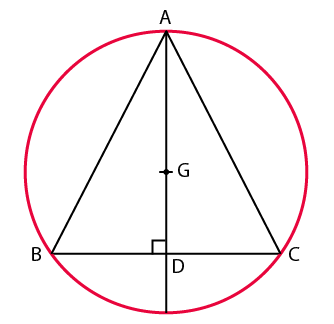Using the Pythagoras theorem

Substituting the values

On further calculation

So we get

By taking out the square root

We know that the centroid and circumcenter coincide in a equilateral triangle

AG: GD = 2: 1

The radius can be written as

By substituting the values

AG = (2/3) × (9√3/ 2)

So we get

AG = 3√3 cm

Therefore, the radius of the circle is 3√3 cm.

21. In the adjoining figure, AB and AC are two equal chords of a circle with centre O. Show that O lies on the bisector of ∠BAC.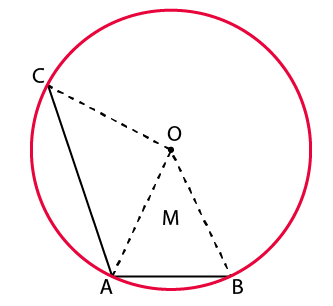Solution:

Consider △ OAB and △ OAC

It is given that AB = AC

OA is common i.e. OA = OA

From the figure we know that OB = OC which is the radii

By SSS congruence criterion

△ OAB ≅ △ OAC

∠OAB = ∠OAC (c. p. c. t)

Therefore, it is proved that O lies on the bisector of ∠BAC.

22. In the adjoining figure, OPQR is a square. A circle drawn with centre O cuts the square in X and Y. Prove that QX = QY.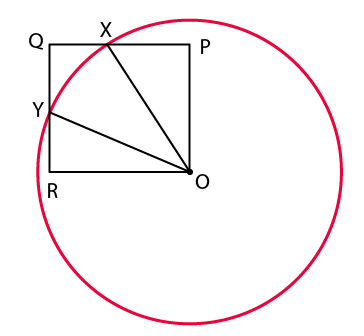Solution:

Consider △ OXP and △ OYR

We know that ∠OPX and ∠ORY are right angles

So we get

∠OPX = ∠ORY = 90o

We know that OX and OY are the radii

OX = OY

From the figure we know that the sides of a square are equal

OP = OR

By RHS congruence criterion

△ OXP ≅ △ OYR

PX = RY (c. p. c. t)

We know that PQ = QR

So we get

PQ – PX = QR – RY

QX = QY

Therefore, it is proved that QX = QY.

23. Two circles with centres O and O’ intersect at two points A and B. A line PQ is drawn parallel to OO’ through A or B, intersecting the circles at P and Q. Prove that PQ = 2OO’.

Solution:

Construct OM ⊥ PQ and O’N ⊥ PQ

So we get

OM ⊥ AP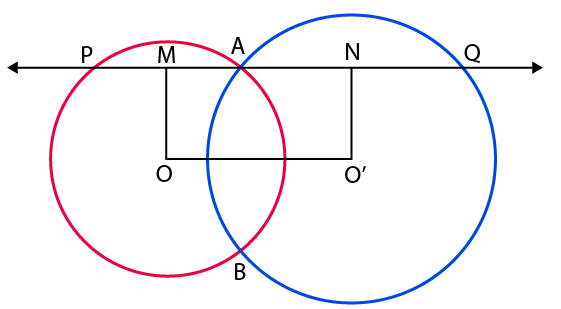We know that the perpendicular from the centre of a circle bisects the chord

AM = PM

It can be written as

AP = 2AM ………. (1)

We know that

O’N ⊥ AQ

We know that the perpendicular from the centre of a circle bisects the chord

AN = QN

It can be written as

AQ = 2AN ……. (2)

So we get

PQ = AP + PQ

By substituting the values

PQ = 2 (AM + AN)

We get

PQ = 2MN

From the figure we know that MNO’O is a rectangle

PQ = 2OO’

Therefore, it is proved that PQ = 2OO’.

### Access other exercise solutions of Class 9 Maths Chapter 12: Circles

Exercise 12B Solutions 18 Questions

Exercise 12C Solutions 26 Questions

### RS Aggarwal Solutions Class 9 Maths Chapter 12 – Circles Exercise 12A

RS Aggarwal Solutions Class 9 Maths Chapter 12 Circles Exercise 12A mainly consists of theorems based on congruence and chord properties of various types of circles.

### Key features of RS Aggarwal Solutions for Class 9 Maths Chapter 12: Circles Exercise 12A

• The students can use the PDF of solutions as a reference material to solve problems according to CBSE guidelines.
• The solutions are prepared by knowledgeable experts to help students understand concepts faster.
• The PDF can be used as a vital resource by the students for revision and exam preparation.
• The exercise wise problems are designed in such a way they will help students to solve problems in a shorter duration.